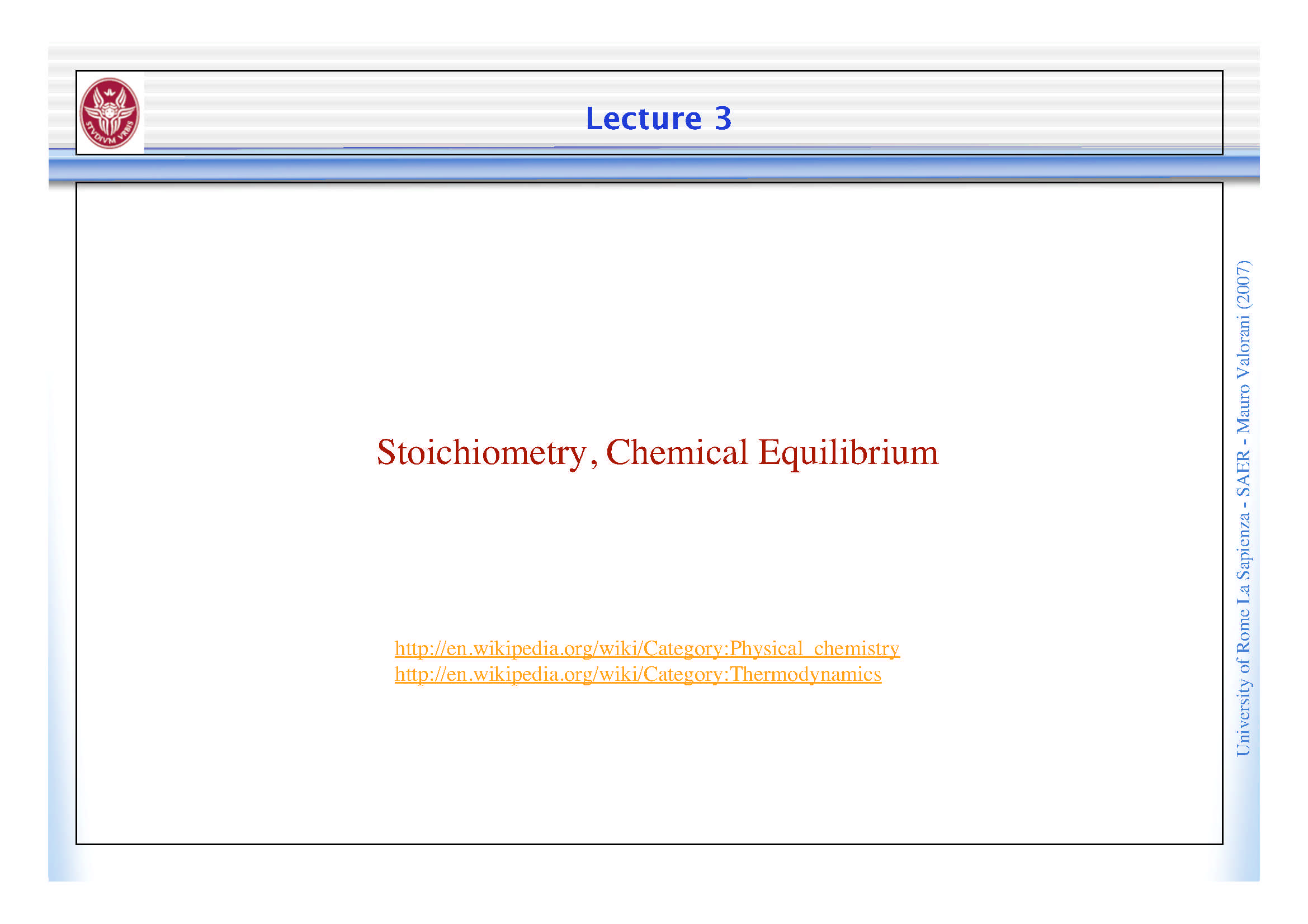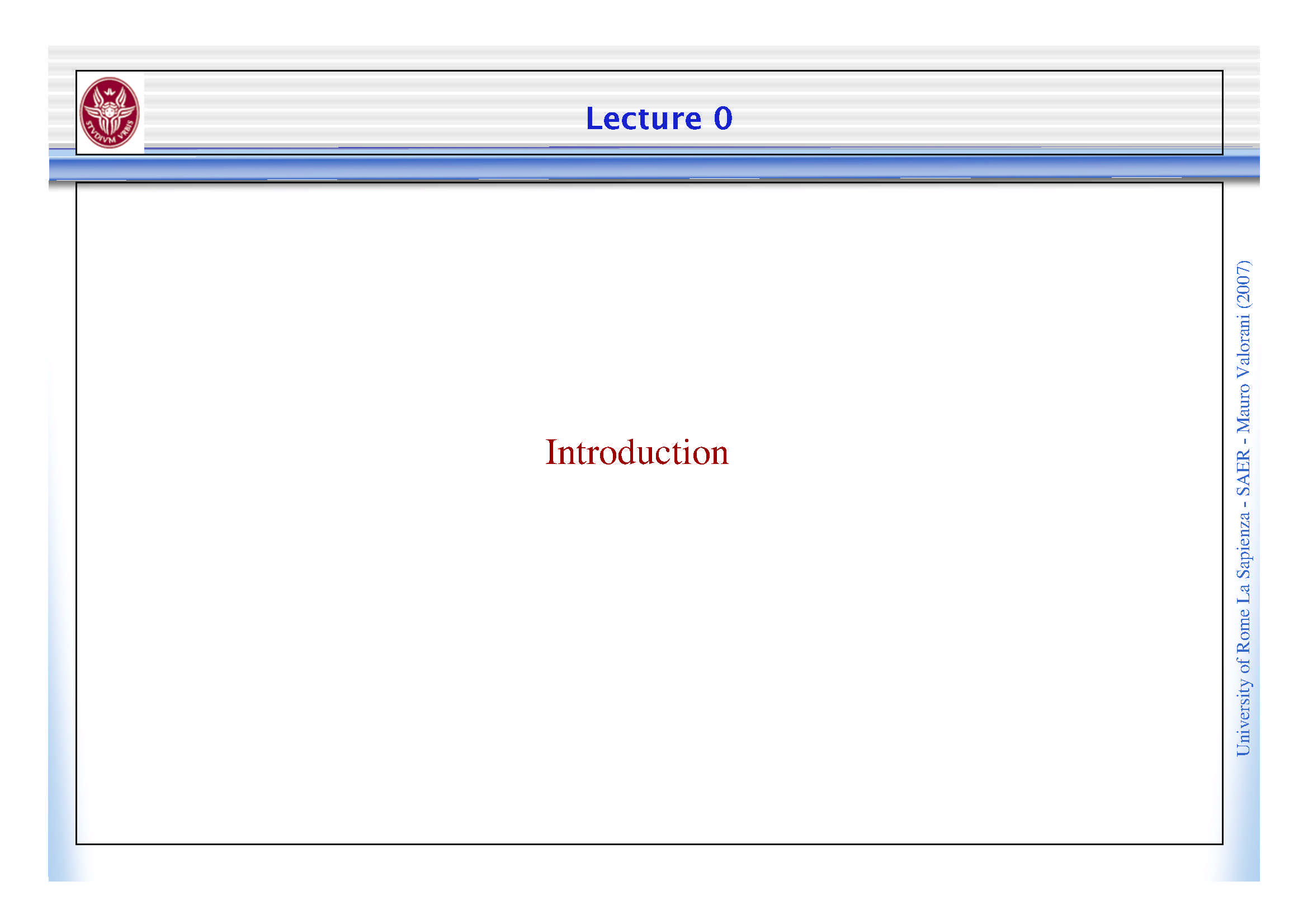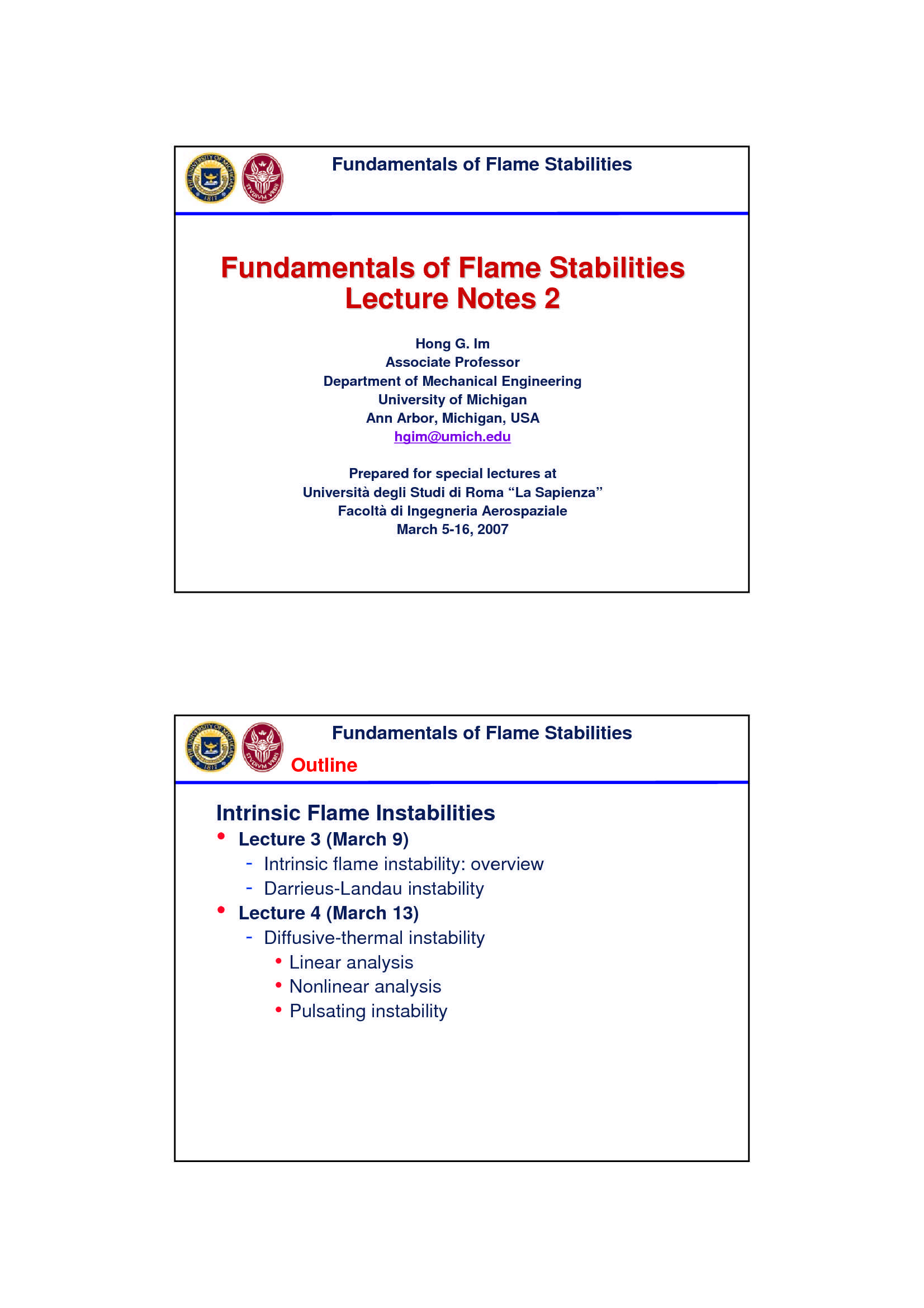Che materia stai cercando?

# Stabilità della fiamma

Materiale didattico per il corso di Combustione del Prof. Mauro Valorani, all'interno del quale sono affrontati i seguenti argomenti: fondamenti di stabilità della fiamma; limiti di stabilità della fiamma; numero di Zeldovich; la distanza di spegnimento; stabilizzazione della fiamma e stabilizzazione del bruciatore; soluzioni di similarità,... Vedi di più

Esame di Combustione docente Prof. M. Valorani

Anteprima

### ESTRATTO DOCUMENTO

Fundamentals of Flame Stabilities

• Theoretical Analysis: 1D Flame with Heat Loss

Basic Flame Structure

( )

1

β −∞

ε 2

O 1/

flame

## T

−∞ λ λ β

( ) ( )

ρ ρ

u c u c

λ

−∞ −∞

1

p p

( )

ρ β

u c

−∞ p

Fundamentals of Flame Stabilities

Approximating a linear temperature profile near maximum

⎛ ⎞ T

x

( ) =

⎜ ⎟

T x

⎝ ⎠

−∞ δ R

activation temp

T T / T

e a R

⎡ ⎤

− = − − ∞

T / T ⎢ ⎥

a

exp

e a ( ) δ

− − + δ

/

T T T x

⎣ ⎦

−∞

⎡ ⎤

⎧ ⎫

⎛ ⎞

⎪ ⎪ 1

T T T x ( )

≈ − + <<

= − + +

−∞

⎢ ⎥

⎨ ⎬ 1 y y 1

⎜ ⎟

exp 1 +

δ 1 y

⎪ ⎪

⎢ ⎥

⎝ ⎠

⎩ ⎭

⎣ ⎦

⎛ ⎞ ⎛ ⎞ − −

β

= − − β −∞ −∞

= =

⎜ ⎟ ⎜ ⎟

exp exp δ 2 2

⎝ ⎠

⎝ ⎠

## T T RT

Zeldovich number

δ δ β

⇒ Reaction is confined within a thin layer of ~ /

## R T

Fundamentals of Flame Stabilities

• Theoretical Analysis: 1D Flame with Heat Loss

(Buckmaster & Ludford; Williams, Ch. 8; Law, Ch. 8)

- Single reactant with “volumetric” heat loss

2

d T dT [ ]

λ ρ

− = − − +

exp /

uc Aq Y E RT L

p c

2

dx dx =

## L

where volumetric heat loss (a phenomenological form)

( ) ( )

ρ ρ

=

f u u

= burning rate (eigenvalue)

−∞ ∞

1

ε = small parameter for expansion

β

Fundamentals of Flame Stabilities

• Boundary Conditions

- Downstream

dT = = = ∞

0; Y 0 at x

dx

- Upstream

(a) Freely-propagating flame

= = = −∞

## T T Y Y x

; at

−∞ −∞

(b) Burner-stabilized flame

dT dY

ρ ρ

= = − = = −

; ; at

T T L uY D uY x x

−∞ −∞

s f

dx dx

where the 2nd temperature condition is used to determine .

x f

Fundamentals of Flame Stabilities

Phenomenological Analysis: Energy Balance

For a freely-propagating flame εθ

= −

( )

= −

Q fc T T

−∞

= −

Q fc T T

−∞

out p f

−∞ λ λ

λ / c / c

c

/ p p

− − −

= = l ~

p λ

Q l L L ε

D c

/

f

loss D f + +

=

f p

## Q L

loss f

λ ( )

( ) c

/

( ) − +

− = − + +

p

fc T T fc T T L L

−∞ −∞

Fundamentals of Flame Stabilities

λ

( ) ( )

/ c

( ) − +

− = − + +

p

fc T T fc T T L L

−∞ −∞

Define nondimensional variables

λ / c

= = =

p 0

T c T / q Y , L L , f f / f ,

( )

−∞

p c 2

ε 0

f q Y

−∞

c

such that the nonadiabatic flame temperature is expressed as

θ − +

⇒ = = +

εθ

= − 2

L / f ; L L L

T T v v

⎛ ⎞

c T

= =

⎜ ⎟

where 1 by definition

## T

⎝ ⎠

q Y

−∞

c ( )

O 1

which implies that heat loss of causes the flame

( )

ε

temperature drop of which is sufficient to cause extinction.

## O

Fundamentals of Flame Stabilities

⎛ ⎞

2 2 2

c

## T RT RT

1

From Frank-Kamenetskii results = = =

p

⎜ ⎟

⎜ ⎟

( )

β −

## T E qY E T T

⎝ ⎠

−∞ −∞

( ) ( )

( ) 2 β − − = −

0 2 4

f ~ exp T / T T exp T / T

( ) εθ

− = −

2 4

f ~ T exp T / T with T T

f a f f ad ⎛ ⎞

⎛ ⎞ ⎡ ⎤

θ

( ) T T

4

εθ ε

= − − ≈ − +

⎜ ⎟

2 4

⎜ ⎟

a a ⎢ ⎥

f T T

exp exp 1

⎜ ⎟

εθ

⎝ ⎠ ⎣ ⎦

## T T T

⎝ ⎠

( ) [ ]

2 θ

= −

0

f exp ( )

( ) [ ] θ

2 ⎡ ⎤ =

θ

= = − = − 2

2 0 2 Recall L / f

/ exp exp /

f f f L f

⎣ ⎦ v

v

where the nonlinearity arise from the indirect effect of

convection ( on flame temperature. Thus,

f) = −

2 2

f ln f L

v

Fundamentals of Flame Stabilities

(a) Freely Propagating Flame

= −

2 2

f ln f L

v

volumetric heat loss parameter

f − =

1/ 2

e 0.61 −

1

e

## L

v

Fundamentals of Flame Stabilities

• Fundamental Flammability Limit

Flame extinction when the burning rate is reduced to

φ ⇒

61%, which happens at flammability limits

cr

⎡ ⎤

RR ~ exp E / RT

⎣ ⎦ Since a roughly fixed rate of

f heat loss may be expected in

a given configuration, the φ

strong variation in RR with

Critical RR can be responsible for the

weak dependences of the

limits on specific experimental

T f configurations.

(Liñán & Williams, p. 97)

0 0.5 1.0 1.5 2.0

φ

Fundamentals of Flame Stabilities

• Kinetic Criterion for Flammability Limits (Law, Ch. 8)

For lean hydrocarbon & lean/rich hydrogen flames

Branching (B): H + O OH + O

2 → +

Termination (T): H + O + M HO M

2 2

Relative sensitivity exponent:

φ

∂ ∂ ∂

w w

ln / ln ln

( )

α φ = =

φ

∂ ∂ ∂

w w

ln / ln ln

## B B

α =

riterion: 1

Kinetic flammability c

Methane/air flame at 1 atm:

φ φ

= =

cr cr φ

which also reflects the fact that RR is very sensitive to variation.

Fundamentals of Flame Stabilities

Quenching Distance

- Due to conductive loss to the solid wall

- Rule of thumb:

Loss 1

≈ β

Generation ( ) ( )

β

: T drop by O 1/ causes RR drop by O 1

Conduction to the wall: δ

Δ

( ) ( )

λ π δ πλδ

≈ = Δ

2

D /2

Generation: D

π

⎛ ⎞

2

## D

ρ

≈ Δ = Δ

⎜ ⎟

Q mc T S c T

p u L p

⎝ ⎠

4

Fundamentals of Flame Stabilities

Quenching criterion: ( ) ⎛ ⎞

πλδ Δ ⎛ ⎞

λ δ

2

L 1

= = ⎜ ⎟ ⎜ ⎟

8 ⎜ ⎟

ρ

π

⎛ ⎞ 2

2 ⎝ ⎠

Q c S D

⎝ ⎠

## D

ρ Δ u p L

⎜ ⎟

S c T

u L p

⎝ ⎠

4

α λ

δ α =

u

Considering that ~ ; ρ

u

S c

L u p

α 2

8 S D

1

L ( )

β β

= ≈ ⇒ ≈ ≈ ≈

u L 8 9 10

β α

2 2

## Q S D

L u

Peclet number ≈

More rigorous analysis (Ronney et al.) yields Pe 60 at quenching.

α

60 1/ 1

p

= ∼

u ~ (be careful at high pressure!)

D −

q 1 / 2

n

S p p

## L

Fundamentals of Flame Stabilities

(b) Burner-Stabilized Flame

=

Consider 0 (no volumetric heat loss)

## L

v ≠

Heat loss is only to the burner ( 0)

s

2

d T dT [ ]

λ ρ

− = − −

exp /

uc Aq Y E RT

p c

2

dx dx

2

d Y dY [ ]

ρ ρ

− = −

exp /

## D uc AY E RT

p

2

dx dx

Boundary Conditions: dT dY

ρ ρ

+

= = = − =

0 : ; ;

x T T L uY D uY

−∞

s s

dx dx

dT dY

( )

= ∞ = < = = =

: ; 0; 0

## x T T T Y

Fundamentals of Flame Stabilities

Nondimensional Equations 0 cT

f Y

= = = p

x x Y T

Define non-D variables: ; ;

λ c Y q Y

/ −∞ −∞

p c

λ

= =

and assuming Le 1 to form S-Z coupling function:

ρ c D

p

( ) ( )

2

d d

+ − + =

f T Y T Y 0

2

dx dx

Boundary Conditions: dT dY

+

= = = − =

0 : ; ;

x T T L fY f

s s

dx dx

dT dY

= ∞ = = = =

: ; 0; 0

x T T Y

f dx dx

Fundamentals of Flame Stabilities

Schematic of Burner-Stabilized Flame

=

s <

−∞ f

=

−∞

⎛ ⎞

dT

λ

= ⎜ ⎟

## L

s ⎝ ⎠

dx +

=

at burner surface

= x

x 0 f

Fundamentals of Flame Stabilities

The Solution: ( )

⎡ ⎤

+ − − =

f T T T L fT

⎣ ⎦

−∞

( )

⎛ ⎞ f T T

dT

= = f s

⎜ ⎟

L ( )

s −

⎝ ⎠

dx fx

exp 1

+

= f

x 0 ⎡ ⎤

4

⎛ ⎞ ⎛ ⎞

T 1 1

= − −

⎢ ⎥

⎜ ⎟ ⎜ ⎟

f

2

f T

exp

⎜ ⎟ ⎜ ⎟

a

⎢ ⎥

## T T T

⎝ ⎠ ⎝ ⎠

⎣ ⎦

For a given mass burning rate, f ,

we can determine , and .

T L x

f s f

Fundamentals of Flame Stabilities

(b) Burner-Stabilized Flame T / T

Dual solutions are both physical!

Fundamentals of Flame Stabilities

Burner-Stabilized Flame Experiment little heat loss

flame as strong

but unstable

flame weakens

feeble flame less heat loss

by heat loss

unstable stronger flame

and lifts up blown away

minimum height

## Q Q Q Q

loss

loss loss loss loss

cooled

porous plug

ṁ ṁ ṁ ṁ ṁ

Fundamentals of Flame Stabilities

Flame Stabilization at Burner Rim

Premixed Flame Stabilization at Burner Rim

- Bunsen Burner Tip (singularity)

Tip (singularity) Mixing layer

? boundary

α U α

=

S U sin

L Heat loss

## U U

Ideal Real

Uniform flow Parabolic flow

Fundamentals of Flame Stabilities

Flame Stabilization at Burner Rim

Flashback Mechanism (Lewis & von Elbe, 1987)

## S D

Blow-off = L q

Velocity Recall Pe ,

Stablized α

Flashback u

2

dU ≈ =

## L L

α

60

dr D

q u

⎧ −

1

0 130 s (stoich. HC-air)

S ≈⎨

L Flame speed −

1

500 s (actual experiment)

For Poiseuille flow

4

dU U

=

dr R

=

D r R

Distance from burner wall

q

How can the stabilization point be so sensitive?

(only one value of velocity)

PAGINE

23

PESO

541.76 KB

AUTORE

PUBBLICATO

+1 anno fa

### DESCRIZIONE DISPENSA

Materiale didattico per il corso di Combustione del Prof. Mauro Valorani, all'interno del quale sono affrontati i seguenti argomenti: fondamenti di stabilità della fiamma; limiti di stabilità della fiamma; numero di Zeldovich; la distanza di spegnimento; stabilizzazione della fiamma e stabilizzazione del bruciatore; soluzioni di similarità, numero di Schmidt.

DETTAGLI
Esame: Combustione
Corso di laurea: Corso di laurea magistrale in ingegneria aeronautica
SSD:
A.A.: 2007-2008

I contenuti di questa pagina costituiscono rielaborazioni personali del Publisher Atreyu di informazioni apprese con la frequenza delle lezioni di Combustione e studio autonomo di eventuali libri di riferimento in preparazione dell'esame finale o della tesi. Non devono intendersi come materiale ufficiale dell'università La Sapienza - Uniroma1 o del prof Valorani Mauro.

Acquista con carta o conto PayPal

Scarica il file tutte le volte che vuoi

Paga con un conto PayPal per usufruire della garanzia Soddisfatto o rimborsato

RecensioniTi è piaciuto questo appunto? Valutalo!

## Altri appunti di CombustioneDispensa

### Stechiometria ed equilibrio chimicoDispensa

### Combustione omogenea e combustione eterogeneaDispensa

### Instabilità della fiammaDispensa